Norons
Share
Explore#What is this?What is this?

This is an exploration of an alternative machine learning model. It’s a different mechanism to neural networks and transformers. If you’re an actual researcher, just know that I haven’t figured out the gradient descent for it, so it’s not really useful yet.
Neural networks are based on interconnected neurons that “feed information forward” through various layers of nodes.
Here’s the best explanation on the internet, this is for a convolutional neural network (CNN) but the main idea transfers well to other places.
In place of neural networks, transformers have become popular—especially for understanding natural language.
In short, this is because natural language can’t be “averaged” in the same way that images can. If you hide some parts of this image:You’ll still understand that these are cute puppies.
But if you have the sentence:
and you drop the word “not”, you’re going to get a completely incorrect meaning.
Transformers are great at paying attention to all of the words. You can read about how they work here:
Without too much detail, the summary is that over time the transformer learns the “shape” of questions to ask whenever it encounters some word. For example, if it encounters the word ‘not’ it might know to ask questions of the shape “not what?”.
I call this a shape because transformers frame that question in a very mathy way—by using a list of numbers called a vector. But we don’t need to go into the details, because my main goal is to compare transformers with what I’m playing with in this doc.
Transformers take a list of words (or “tokens”) and sees how they relate to one another. Over time, attention given to an unimportant token is atrophied until only the tokens that matter remain.

A noral net does something similar, but it focuses on propagating signals that say, “don’t pay attention to this.” Those signals are really aggressive, if they get triggered they basically cut out whole sections of the network. This is nice because instead of our weights being diffusely dispersed across our entire network, we should end up with fairly modularized functionality. In other words, it acts a bit like a decision tree, but it learns aggregate criteria instead of making decisions only based on discrete features. The hope is that this will have some increased interpretability, as well as better robustness to overfitting.

In case it’s not already clear, this is all speculative and should be treated with the same level of deference as you would any other idea generated by someone larping at being an ml researcher.

# How does it work?

It works by using nested NOR gates and a signaling protocol.
What’s a nor gate? It’s a type of gate used in boolean logic and electrical engineering that can be used to express any computation.
If you wanted, you could build an entire computer that ran off of only these little guys.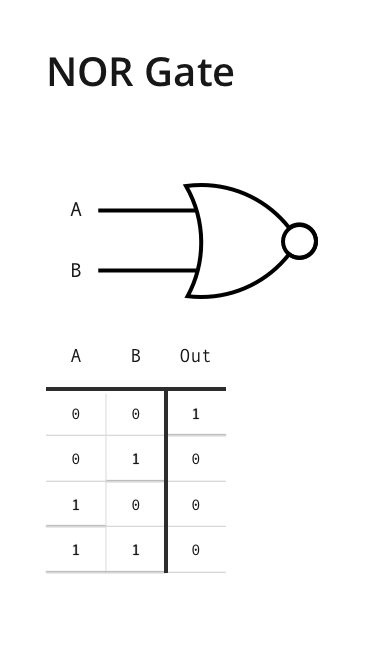Essentially what that diagram says is that the only time you get an output signal of 1 (an “on” signal) is if you put in two signals of 0 (or “off”) signals, one 0 signal on line A and the other 0 signal on line B.
Here’s a wonderful video that includes a very cute cat which explains gates:
There’s a way to generalize the NOR gate beyond just two inputs. We can make it accept 3, 4, or more inputs. We might want to do this if there are more than two signals coming into the noron that we want to pay attention to. Here’s what it’s like with 3 inputs: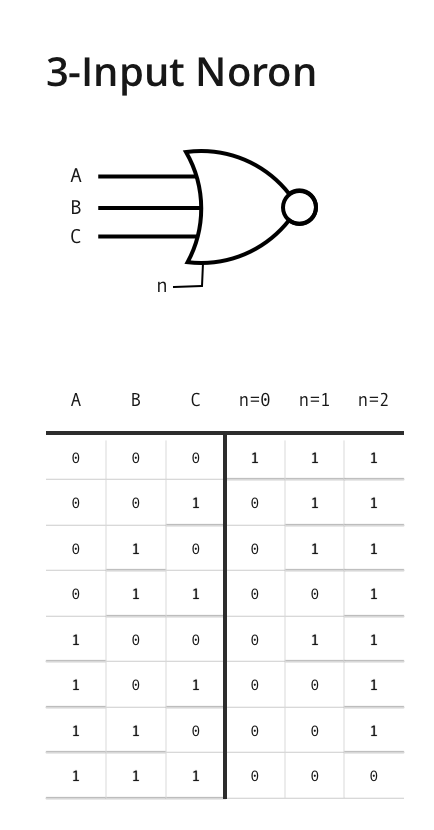You can see that once we have three input gates we need another variable (I called it n here) that lets us select what “mode” we want to be in.
In mode n=0 (see column above) we ignore most everything, only A=0, B=0, and C=0 result in a 1 output, everything else is 0.
In mode n=2 we accept almost everything, only A=1, B=1, and C=1 result in a 0 output, everything else is 1.
A simple way to think about what this is saying is:
When n equals some number, only that many incoming signals can be high if you want a high output.
So n = 0 gives you 1 as the output only when all of A,B, and C are 0.
But n = 1 gives you 1 whenever no more than one of A, B, or C is 1. etc.
Now that we have it with 3 inputs what if we try more?
Here we generalize to as many input signals as you want.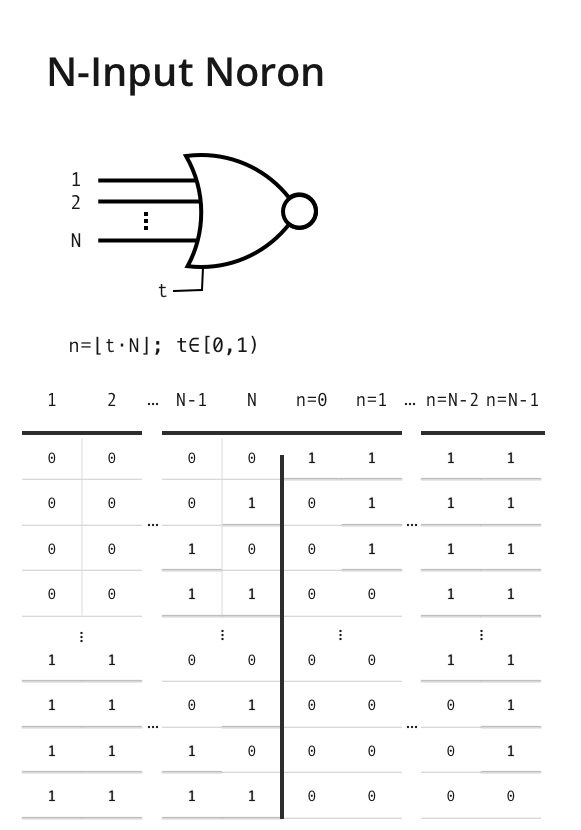This might look intense, but it’s the same as before, just with however many input signals we want (now the inputs use numbers 1, 2, ...).
The other thing that changes is that we’re now using t to find our n. We do this so we can compare how sensitive norons are to each other no matter how many inputs n they might have.
For example, if t = .5 and the noron has 4 inputs, we give it n = 2.
But, if t = .5 and the noron has 50 inputs, we give it n = 25. If we were just comparing n then these two would look really different, 25 vs 2, but if we compare t instead they look identical because t = .5 in both cases.
In general, if number of input signals =
000
96
and t =
0000
0.24
then n =
23
. This can also be written as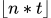The smaller t is the more sensitive it is, meaning the more easily it will output a 0. (If you want, you can think about a small t as a gate that’s unexcitable, a big t as a gate that’s always ecstatic).
So far so good. But how do you use this to train a network of these to learn? (Spoiler, I don’t know yet. That’s why I made this doc, but I have some ideas).
What we’ll do is we’ll implement a simple algorithm that has the ability to ignore signals. If the signal value on the input edge (edge value) is less than the threshold value we specify for that edge (edge threshold) then we return a 0 (edge value not big enough), otherwise we return a 1.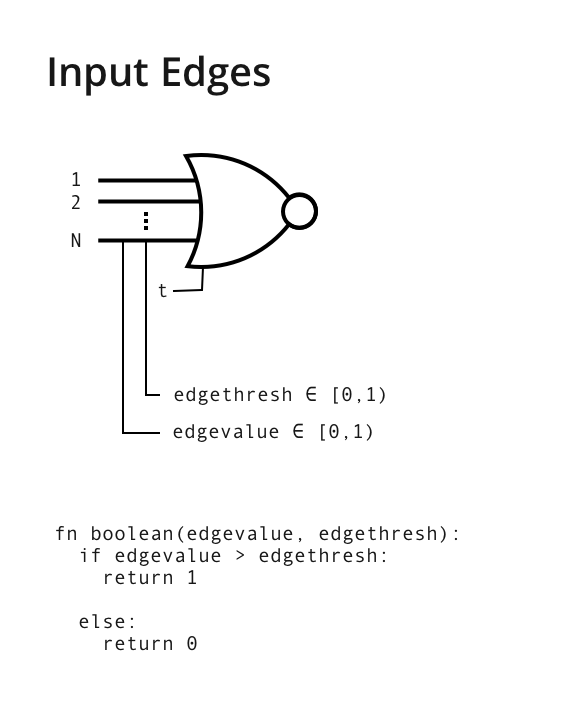I.e. if the edge value is bigger than the threshold the signal is high.
Then you give yourself control over the value of the output edge. Here’s how that would work written in pseudocode (in a made up pseudolanguage):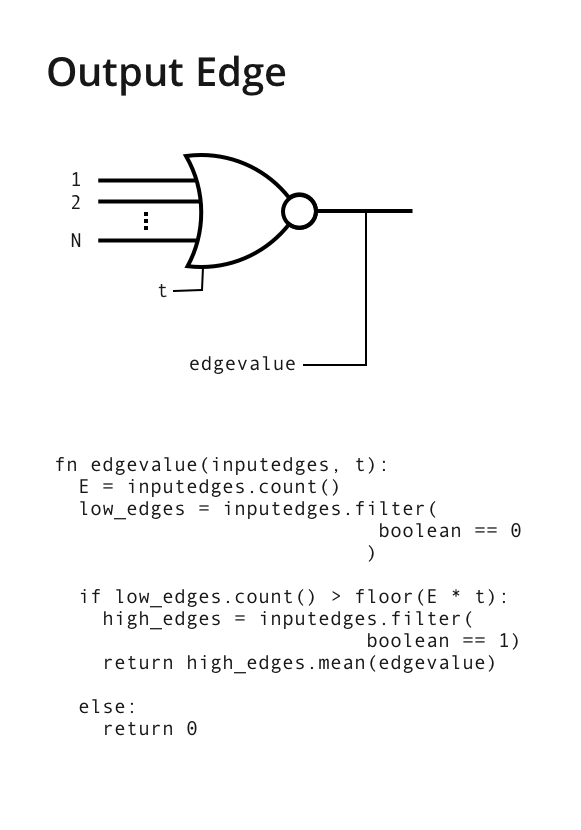It might seem complicated in the above image, but it’s pretty simple. Here’s the same code written in Coda’s formula language, I’ve color coded the steps to match the explanation below:
If( thisRow.Edges.Filter(High? = false).Count() > Floor(thisRow.Edges.Count() * t), thisRow.Edges.Filter(High? = true).Value.Average(), 0)
Basically, calculate the n based on the t and the number of input edges. Then treat n like a nor gate. Remember:
When n equals some number, only that many incoming signals can be high if you want a high output.
So n = 0 gives you 1 as the output only when all of A,B,C are 0.
But n = 1 gives you 1 whenever no more than one of A, B, or C is 1. etc.
But then here’s an interesting choice: if it’s activated then return the average of the high values (High? = true).
If too many edges are low, return 0.
Let’s say we want a noron to output a zero value to the next layer (meaning, “Ignore me!”) here’s how we can achieve that:
Our friendly noron can set really big thresholds for all of the edges, so they tend to be low (High? = false) and therefore don’t get bigger than n. This causes them to return 0.
Or it can set t to a low number like 0. This makes the noron super boring, almost no matter what you throw at it it evaluates to false and returns 0

If it wants the signal that’s passed on to the next layer to be large it can:
Set high thresholds for edges it wants to pass to the next layer, low thresholds for the edges it wants to sacrifice to get the count of low bigger than the value of n
And has to set t to a small enough number so as to make n smaller than the number of low edges.

Here, it’s easier if you play with it.
Try these two exercises:
Set up the below controls so that edge 1 is completely in control of output value (i.e. that no matter what changes happen to the value of edge 2 or edge 3, output value always shows the value of edge 1)
Set it up so that output value is the average of edge 1 and edge 2’s values (hint: you’ll have to change the t value)

0000
0.91
edge 1
threshold
0
0
high?
00.91
0000
0.57
edge 2
threshold
0
0
high?
00.57
0000
0.47
edge 3
threshold
0
0
high?
00.47
noron
input edges
[00.91][00.57][00.47]
t value
0
0
implied n
0
low count
0
low count > n?
×
output value
0

If you press this button 👇 it makes it so that output value is whatever input edge 1 has as its value
Set up so edge 1 has control
now if you change edge 1’s value it will become the output value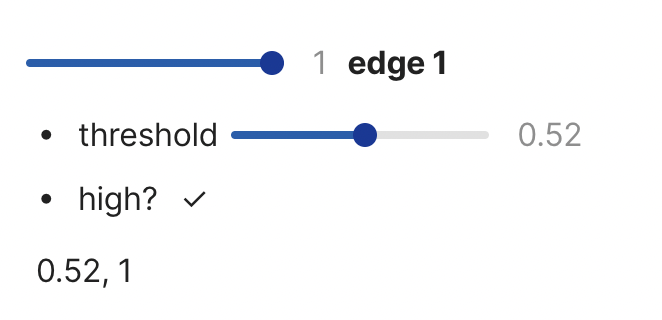This creates an interesting behavior for the system. It kinda has two channels for information in a noron. It pushes some edges low to say, “hey, my neighbors have information, listen up.” and it pushes other information high to say, “this is the stuff worth paying attention to.” It feels like it would tend to put the network in a state, always sacrificing information in order to signal boost other information.
Open question
I suppose you could also invert the current structure. So you could put the signal information (”pay attention”) in the high edges and the value information (”this is the stuff”) in the low edges. Would that make a difference?
My question is this: what kind of protocol could train a network like this? We can’t use directly because it requires a differentiable activation function, whereas our states are discontinuous. Instead, maybe we could:
Train a differentiable model to metalearn the weights for the discontinuous model.
Train a differentiable model that approximates the discontinuous model, then collapse its weights into discontinuous weights.
Bootstrap by having the model learn itself.

You know which one I prefer.
Share